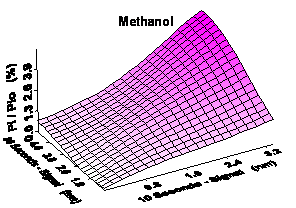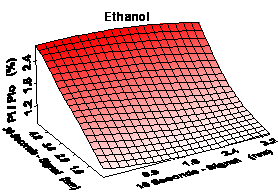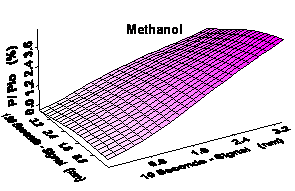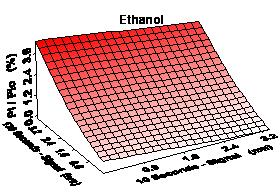Home News About Me Ph. D. Thesis Abstract Table of Contents 1. Introduction 2. Theory  Fundamentals of the Multivariate Data Analysis 3. Theory  Quantification of the Refrigerants R22 and R134a: Part I 4. Experiments, Setups and Data Sets 5. Results  Kinetic Measurements 6. Results  Multivariate Calibrations 7. Results  Genetic Algorithm Framework 8. Results  Growing Neural Network Framework 9. Results  All Data Sets 9.1. Methanol and Ethanol by SPR 9.1.1. Single Analytes 9.1.2. Parallel Growing Neural Network Framework 9.1.3. Sensitivity Analysis 9.1.4. Brute Force Variable Selection 9.1.5. Conclusions 9.2. Methanol, Ethanol and 1-Propanol by SPR 9.3. Methanol, Ethanol and 1-Propanol by the RIfS Array and the 4l Setup 9.4. Quaternary Mixtures by the SPR Setup and the RIfS Array 9.5. Quantification of the Refrigerants R22 and R134a in Mixtures: Part II 10. Results  Various Aspects of the Frameworks and Measurements 11. Summary and Outlook 12. References 13. Acknowledgements Publications Research Tutorials Downloads and Links Contact Search Site Map Print this Page### 9.1.3.   Sensitivity Analysis

The small number of 3 variables allows an efficient sensitivity analysis , to give a little insight into the "black box" of neural networks. Thereby the values of two time points were systematically varied between the measured minimum and maximum sensor responses of these time points with the third time point kept constantly in the middle of the corresponding range of the measured sensor responses. The trained neural nets described above were fed with the values of the 3 time points. The concentrations calculated by the neural nets are plotted versus the two varying time points in figure 62.

The top row of this figure shows the prediction of the concentrations of methanol (left) and ethanol (right) depending on the sensor responses of the time points 10 s (x-axis) and 30 s (y-axis). The prediction of methanol is determined by the ensemble of both time points. This is in accordance with figure 15, which demonstrates that the sorption process of methanol has come to a steady state after 10 s. Hence, a high sensor response after 10 s caused by a high concentration of methanol automatically induces a high sensor response after 30 s. The top right plot of figure 62 shows that the prediction of ethanol is practically not influenced by the time point 10 s explainable by the variance of this time point being mainly caused by the sorption of methanol (see figure 15). Nevertheless, the prediction of ethanol is nearly linearly correlated with the sensor response of the time point 30 s. The bottom row of figure 62 shows the prediction of the concentration depending on the signal of the time points 10 s (x-axis) and 130 s (y-axis). The plane parallel to the y-axis demonstrates that the prediction of methanol is practically independent from the time point 130 s. However, the prediction of ethanol highly depends on the sensor response of the time point 130 s. The plots of figure 62 top right and bottom right are nearly identical except of the higher dynamics of time point 130 s.figure 62:  Predicted concentrations of the sensitivity analysis versus the sensor responses of the two time points varied.

In summary, it may be said that the prediction of methanol depends on the combination of the time points 10 s and 30 s whereas the prediction of ethanol depends on the time points 30 s and on the time points 130 s. The similar dependencies of the predictions of ethanol on the time points 30 s and 130 s indicate that the time point 130 s could be rendered unnecessary by calculating the concentrations of methanol using the sensor response of the time point 10 s and by calculating the concentration of ethanol by the ratio of the sensor responses after 10 s and 30 s. Thus, neural networks of the topology 2-4-1 were trained using only the time points 10 s and 30 s. These small networks perform quite well predicting both analytes with relative errors of only 2.22% and 2.70% (see table 1). The only small deterioration of the predictive ability is overcompensated by the fact that the measurement time can be reduced from 130 s to 30 s. In addition, the shortening of the time with the polymer being exposed to the analyte results in the sorption of less ethanol into the polymers. Therefore, the desorption of ethanol needs less time to be completed additionally shortening the time needed between 2 measurements.

 Page 117 © Frank Dieterle, 03.03.2019